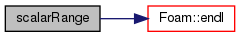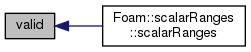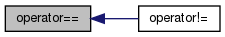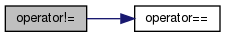The OpenFOAM Foundation
scalarRange Class Reference

A scalar range specifier. More...

## Public Member Functions

scalarRange ()
Construct an empty range. More...

scalarRange (const scalar lower, const scalar upper)
Construct a range from lower to upper. More...

scalarRange (Istream &)
Construct from Istream. More...

bool empty () const
Is the range empty? More...

bool valid () const
Is the range non-empty? More...

bool isExact () const
Is the range 'EXACT'? More...

scalar value () const
The value constituting an 'EXACT' match. More...

scalar lower () const
The lower limit. More...

scalar upper () const
The upper limit. More...

bool selected (const scalar) const
Return true if the value is within the range. More...

bool operator== (const scalarRange &) const

bool operator!= (const scalarRange &) const

static int debug

## Friends

Istreamoperator>> (Istream &, scalarRange &)

Ostreamoperator<< (Ostream &, const scalarRange &)

## Detailed Description

A scalar range specifier.

The range selector can be specified as an "LOWER:UPPER" range, as a "LOWER:" bound, as an ":UPPER" bound or simply as an "EXACT" value. The read constructor uses a colon (:) as a range marker and a comma (,) to delimit the next possible range selector.

Source files

Definition at line 62 of file scalarRange.H.

## ◆ scalarRange() [1/3]

 scalarRange ( )

Construct an empty range.

Definition at line 36 of file scalarRange.C.

## ◆ scalarRange() [2/3]

 scalarRange ( const scalar lower, const scalar upper )

Construct a range from lower to upper.

Definition at line 44 of file scalarRange.C.

## ◆ scalarRange() [3/3]

 scalarRange ( Istream & is )

Construct from Istream.

Since commas can be used as list delimiters, leading and trailing commas are ignored.

Definition at line 59 of file scalarRange.C.

References scalarRange::debug, Foam::endl(), and Foam::Info.

Here is the call graph for this function:## ◆ empty()

 bool empty ( ) const

Is the range empty?

Definition at line 76 of file scalarRange.C.

## ◆ valid()

 bool valid ( ) const

Is the range non-empty?

Definition at line 82 of file scalarRange.C.

Referenced by scalarRanges::scalarRanges().

Here is the caller graph for this function:## ◆ isExact()

 bool isExact ( ) const

Is the range 'EXACT'?

Definition at line 88 of file scalarRange.C.

## ◆ value()

 Foam::scalar value ( ) const

The value constituting an 'EXACT' match.

or the values for 'UPPER' or 'LOWER' limits

Definition at line 94 of file scalarRange.C.

## ◆ lower()

 Foam::scalar lower ( ) const

The lower limit.

Definition at line 100 of file scalarRange.C.

## ◆ upper()

 Foam::scalar upper ( ) const

The upper limit.

Definition at line 112 of file scalarRange.C.

## ◆ selected()

 bool selected ( const scalar value ) const

Return true if the value is within the range.

Definition at line 131 of file scalarRange.C.

## ◆ operator==()

 bool operator== ( const scalarRange & range ) const

Definition at line 156 of file scalarRange.C.

Referenced by scalarRange::operator!=().

Here is the caller graph for this function:## ◆ operator!=()

 bool operator!= ( const scalarRange & range ) const

Definition at line 167 of file scalarRange.C.

References scalarRange::operator==().

Here is the call graph for this function:## ◆ operator>>

 Istream& operator>> ( Istream & , scalarRange & )
friend

## ◆ operator<<

 Ostream& operator<< ( Ostream & , const scalarRange & )
friend

## ◆ debug

 int debug
static

Definition at line 84 of file scalarRange.H.

Referenced by Foam::operator>>(), and scalarRange::scalarRange().

The documentation for this class was generated from the following files: